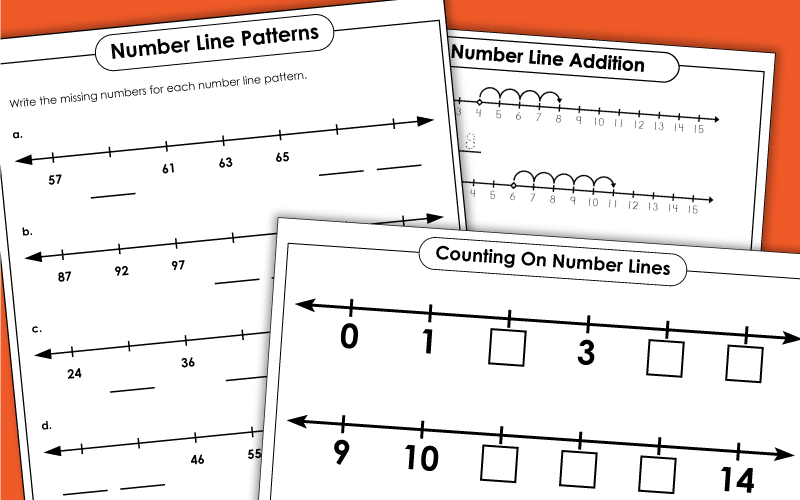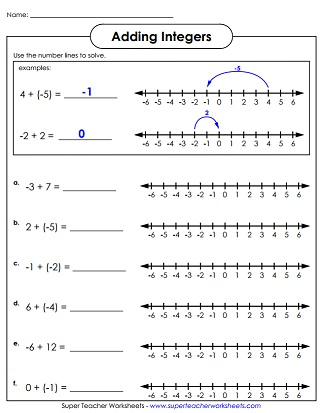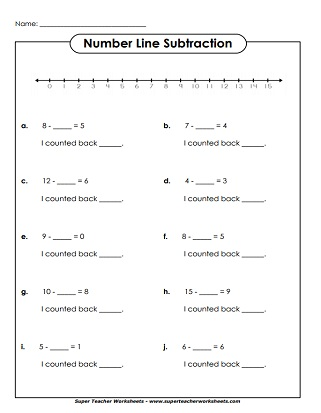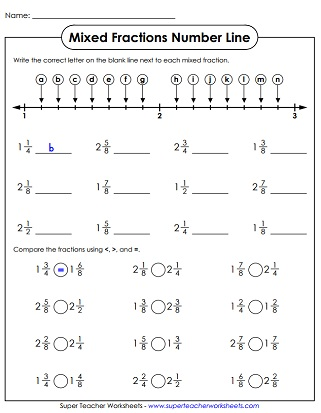# Number Line Worksheets

We have printable number line worksheets for teaching counting, skip counting, addition, subtraction, and number patterns.## Number Lines

Cut out the parts of this number line and glue them together. This number line includes numbers one through ten.
This page has two number lines, with the numbers 0 through 20.
This cut-and-glue number line begins has a range of zero through one hundred. When you attach all of the parts it's about nine feet long!
Cut, glue, and build this giant, 9-foot number line, which includes all integers from negative fifty through positive fifty.

## CountingNumber Lines

Count by ones and write numbers in the empty boxes.
Fill in the missing numbers that come before or after the given numbers on the number lines. This worksheet contains only 2-digit numbers.
Write the numbers that are missing from each row. This page includes only 3-digit numbers.
Complete the number lines with the missing 3-digit numbers.
Write the positive and negative numbers on the number line and answer the questions.

Use the number line to learn and practice basic addition skills.

## Number LineSubtraction

Count backwards on the number line to solve the basic subtraction facts.
Use the number line to solve the problems. Tell how many spaces you had to count back.
Another subtraction worksheet that requires students to count backwards.
Subtract positive and negative integers by counting backward or forward on the number line.
Another worksheet for teaching positive and negative integer subtraction.

## Skip CountingWith Number Lines

Use this number line to count by 2s.  Complete the activity by filling in the boxes.  This number line ranges from 0 to 32.
Count by 3s on these number lines.  The number line begins at 0 and ends at 33.
Skip count by 4s and write the correct numbers in the boxes.
On this page, students will review counting by 10s with a number line.
Counting by twenty-fives is easy to understand with these printable number lines.

## Number LinePatterns

Complete each number pattern on the number lines shown.
Write the missing numbers from each set. Then explain the rule that you followed to determine the answer.
Each number line contains two variables. Write the correct number for each variable.

## Fractions and DecimalNumber Lines

Tell what fraction is represented by each letter on the number lines.
Write the letter from the number line that corresponds with each decimal number.
Each decimal (hundredths) corresponds with a letter on the number line. Match the letters with the numbers.
Tell what decimal is represented by each letter. Decimals are all written to the nearest tenth.

## Double Number LinesFractions, Decimals, Percents

Use the double number line to determine the compare fractions to percents. Use the symbols <, >, and =. (This is an advanced-level worksheet recommended for grades 4 and up.)
Math Worksheets

Super Teacher Worksheets has a huge collection of math worksheets for most skills taught in the elementary grades.

Number Pattern Worksheets

Complete the number patterns and write the rule for each.

Skip Counting Worksheets

Practice skip counting by 2s, 3s, 4s, 5s, 10s, 25s, and 100s.

Hundreds Charts

Hundreds charts can also be used to teach place value, adding, subtracting, skip counting, and number patterns.

## Pictures of Our WorksheetsMy Account
Site Information